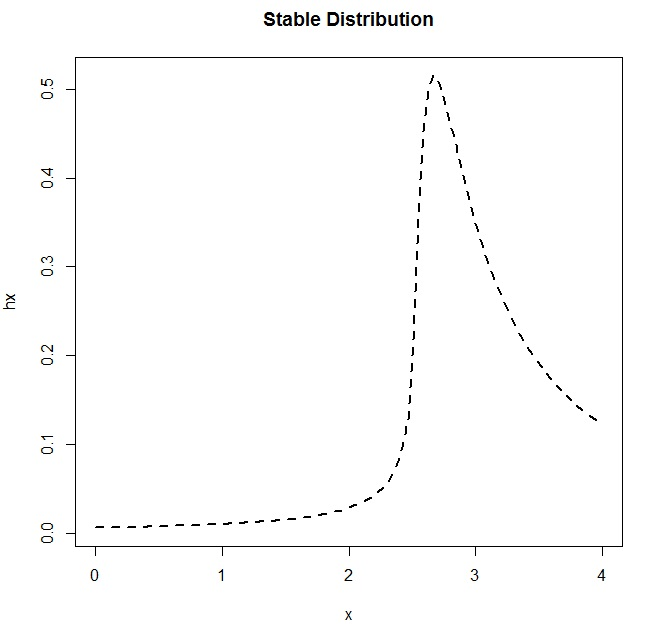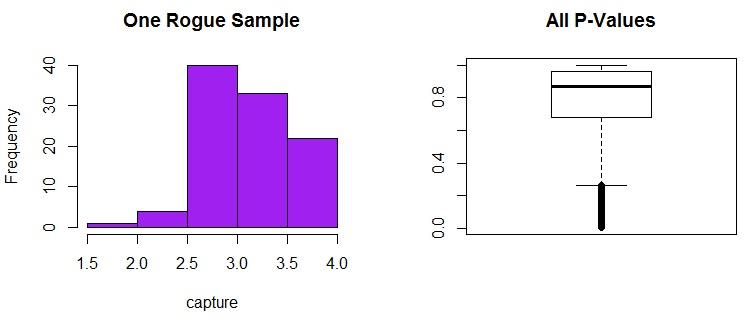## Why the Ban on P-Values? And What Now?

Just recently, the editors of the academic journal Basic and Applied Social Psychology have decided to ban p-values: that’s right, the nexus for inferential decision making… gone! This has created quite a fuss among anyone who relies on significance testing and p-values to do research (especially those, presumably, in social psychology who were hoping to submit a paper to that journal any time soon). The Royal Statistical Society even shared six interesting letters from academics to see how they felt about the decision.

These letters all tell a similar story: yes, p-values can be mis-used and mis-interpreted, and we need to be more careful about how we plan for — and interpret the results of — just one study! But not everyone advocated throwing out the method in its entirety. I think a lot of the issues could be avoided if people had a better gut sense of what sampling error is… and how easy it is to encounter (and as a result, how easy it can be to accidentally draw a wrong conclusion in an inference study just based on sampling error). I wanted to do a simulation study to illustrate this for my students.

The Problem

You’re a student at a university in a class full of other students. I tell you to go out and randomly sample 100 students, asking them what their cumulative GPA is. You come back to me with 100 different values, and some mean value that represents the average of all the GPAs you went out and collected. You can also find the standard deviation of all the values in your sample of 100. Everyone has their own unique sample.

“It is misleading to emphasize the statistically significant findings of any single team. What matters is the totality of the evidence.” – John P. A. Ioannidis in Why Most Published Research Findings are False

It’s pretty intuitive that everyone will come back with a different sample… and thus everyone will have a different point estimate of the average GPA that students have at your university. But, according to the central limit theorem, we also know that if we take the collection of all the average GPAs and plot a histogram, it will be normally distributed with a peak around the real average GPA. Some students’ estimates will be really close to the real average GPA. Some students’ estimates will be much lower (for example, if you collected the data at a meeting for students who are on academic probation). Some students’ estimates will be much higher (for example, if you collected the data at a meeting for honors students). This is sampling error, which can lead to incorrect inferences during significance testing.

Inferential statistics is good because it lets us make decisions about a whole population just based on one sample. It would require a lot of time, or a lot of effort, to go out and collect a whole bunch of samples. Inferential statistics is bad if your sample size is too small (and thus you haven’t captured the variability in the population within your sample) or have one of these unfortunate too-high or too-low samples, because you can make incorrect inferences. Like this.

The Input Distribution

Let’s test this using simulation in R. Since we want to randomly sample the cumulative GPAs of students, let’s choose a distribution that reasonably reflects the distribution of all GPAs at a university. To do this, I searched the web to see if I could find data that might help me get this distribution. I found some data from the University of Colorado Boulder that describes GPAs and their corresponding percentile ranks. From this data, I could put together an empirical CDF, and then since the CDF is the integral of the PDF, I approximated the PDF by taking the derivatives of the CDF. (I know this isn’t the most efficient way to do it, but I wanted to see both plots):

```score <- c(.06,2.17,2.46,2.67,2.86,3.01,3.17,3.34,3.43,3.45,
3.46,3.48,3.5,3.52,3.54,3.56,3.58,3.6,3.62,3.65,3.67,3.69,
3.71,3.74,3.77,3.79,3.82,3.85,3.88,3.91,3.94,3.96,4.0,4.0)
perc.ranks <- c(0,10,20,30,40,50,60,70,75,76,77,78,79,80,81,
82,83,84,85,86,87,88,89,90,91,92,93,94,95,96,97,98,99,100)
fn <- ecdf(perc.ranks)
xs <- score
ys <- fn(perc.ranks)
slope <- rep(NA,length(xs))
for (i in 2:length(xs)) {
slope[i] <- (ys[i]-ys[i-1])/(xs[i]-xs[i-1])
}
slope <- 0
slope[length(xs)] <- slope[length(xs)-1]```

Then, I plotted them both together:

```par(mfrow=c(1,2))
plot(xs,slope,type="l",main="Estimated PDF")
plot(xs,ys,type="l",main="Estimated CDF")
dev.off()```

I looked around for a distribution that might approximate what I saw. (Yes, I am eyeballing.) I found the Stable Distribution, and then played around with the parameters until I plotted something that looked like the empirical PDF from the Boulder data:

```x <- seq(0,4,length=100)
hx <- dstable(x, alpha=0.5, beta=0.75, gamma=1, delta=3.2)
plot(x,hx,type="l",lty=2,lwd=2)```The Simulation

First, I used pwr.t.test to do a power analysis to see what sample size I needed to obtain a power of 0.8, assuming a small but not tiny effect size, at a level of significance of 0.05. It told me I needed at least 89. So I’ll tell my students to each collect a sample of 100 other students.

Now that I have a distribution to sample from, I can pretend like I’m sending 10,000 students out to collect a sample of 100 students’ cumulative GPAs. I want each of my 10,000 students to run a one-sample t-test to evaluate the null hypothesis that the real cumulative GPA is 3.0 against the alternative hypothesis that the actual cumulative GPA is greater than 3.0. (Fortunately, R makes it easy for me to pretend I have all these students.)

```sample.size <- 100
numtrials <- 10000
p.vals <- rep(NA,numtrials)
gpa.means <- rep(NA,numtrials)
compare.to <- 3.00
for (j in 1:numtrials) {
r <- rstable(n=1000,alpha=0.5,beta=0.75,gamma=1,delta=3.2)
meets.conds <- r[r>0 & r<4.001]
my.sample <- round(meets.conds[1:sample.size],3)
gpa.means[j] <- round(mean(my.sample),3)
p.vals[j] <- t.test(my.sample,mu=compare.to,alternative="greater")\$p.value
if (p.vals[j] < 0.02) {
# capture the last one of these data sets to look at later
capture <- my.sample
}
}```

For all of my 10,000 students’ significance tests, look at the spread of p-values! They are all over the place! And there are 46 students whose p-values were less than 0.05… and they rejected the null. One of the distributions of observed GPAs for a student who would have rejected the null is shown below, and it looks just fine (right?) Even though the bulk of the P-Values are well over 0.05, and would have led to the accurate inference that you can’t reject the null in this case, there are still plenty of values that DO fall below that 0.05 threshold.

```> summary(p.vals)
Min. 1st Qu. Median Mean 3rd Qu. Max.
0.005457 0.681300 0.870900 0.786200 0.959300 1.000000
> p.vals.under.pointohfive <- p.vals[p.vals<0.05]
> length(p.vals.under.pointohfive)
 46```
```> par(mfrow=c(1,2))
> hist(capture,main="One Rogue Sample",col="purple")
> boxplot(p.vals,main="All P-Values")```Even though the p-value shouted “reject the null!” from this rogue sample, a 99% confidence interval shows that the value I’m testing against… that average cumulative GPA of 3.0… is still contained within the confidence interval. So I really shouldn’t have ruled it out:

```> mean(capture) + c(-1*qt(0.995,df=(sample.size-1))*(sd(capture)/sqrt(sample.size)),
+ qt(0.995,df=(sample.size-1))*(sd(capture)/sqrt(sample.size)))
 2.989259 3.218201
> t.test(capture,mu=compare.to,alternative="greater")\$p.value
 0.009615011```

If you’re doing real research, how likely are you to replicate your study so that you know if this happened to you? Not very likely at all, especially if collecting more data is costly (in terms of money or effort). Replication would alleviate the issues that can arise due to the inevitable sampling error… it’s just that we don’t typically do it ourselves, and we don’t make it easy for others to do it. Hence the p-value controversy.

What Now?

What can we do to improve the quality of our research so that we avoid the pitfalls associated with null hypothesis testing completely, or, to make sure that we’re using p-values more appropriately?

• Make sure your sample size is big enough. This usually involves deciding what you want the power of the test to be, given a certain effect size that you’re trying to detect. A power of 0.80 means you’ll have an 80% chance of detecting an effect that’s actually there. However, knowing what your effect size is prior to your research can be difficult (if not impossible).
• Be aware of biases that can be introduced by not having a random enough or representative enough sample.
• Estimation. In our example above we might ask “How much greater than 3.0 is the average cumulative GPA at our university?” Check out Geoff Cummings’ article entitled “The New Statistics: Why and How” for a roadmap that will help you think more in terms of estimation (using effect sizes, confidence intervals, and meta-analysis).
• Support open science. Make it easy for others to replicate your study. If you’re a journal reviewer, consider accepting more articles that replicate other studies, even if they aren’t “novel enough”.

I am certain that my argument has holes, but it seems to be a good example for students to better embrace the notion of sampling error (and become scared of it… or at least more mindful). Please feel free to suggest alternatives that could make this a more informative example. Thank you!

• Alan ParkerI think a key point missing from the “let’s ban p” discussion – as far as I’ve seen it so far – is that made, ad nauseum, in “The Cult of Statistical Significance”: statstical significance is much less interesting than effect size. In the real world we always want to know how big an effect is. If the effect is tiny and highly significant, we usually don’t care much. If it is huge and insignificant then it is worth exploring further. So just quoting p without giving the effect size is leaving out the most useful piece of information.

• Pingback: Distilled News | Data Analytics & R

• Arma DilloMost people interpret p-values directly (ala Fisher) and that use of it isn’t consistent with the very idea of frequentism. Dienes 2008 has a really nice, very readable overview of this: http://www.lifesci.sussex.ac.uk/home/Zoltan_Dienes/inference/

• Chas BrodThe fact that, about 80 years after it was introduced, the p-value is so widely mis-understood, and mis-applied by researchers across all disciplines is reason enough to ban the p-value. It was nice, it helped consolidate our thoughts about significance, but we now have better ideas and ways of measuring the idea of significance, so let’s move on now.

• ingorohlfingThe post is nice, but misses the point of the journal’s ban. The editors ban p-values because they are often misinterpreted, not because the editors have fundamental problems with the idea of sampling error. This is not an argument for the ban because I think it goes too far, but the correct interpretation of p-values needs to be enforced by journal editors.